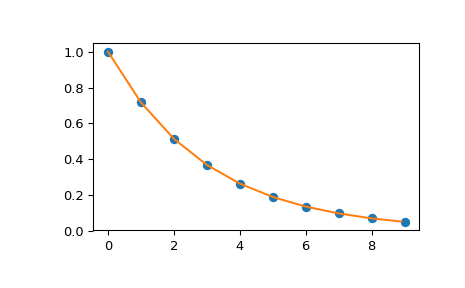# scipy.interpolate.interp1d¶

class scipy.interpolate.interp1d(x, y, kind='linear', axis=-1, copy=True, bounds_error=None, fill_value=nan, assume_sorted=False)[source]

Interpolate a 1-D function.

x and y are arrays of values used to approximate some function f: y = f(x). This class returns a function whose call method uses interpolation to find the value of new points.

Note that calling interp1d with NaNs present in input values results in undefined behaviour.

Parameters: x : (N,) array_like A 1-D array of real values. y : (...,N,...) array_like A N-D array of real values. The length of y along the interpolation axis must be equal to the length of x. kind : str or int, optional Specifies the kind of interpolation as a string (‘linear’, ‘nearest’, ‘zero’, ‘slinear’, ‘quadratic’, ‘cubic’ where ‘zero’, ‘slinear’, ‘quadratic’ and ‘cubic’ refer to a spline interpolation of zeroth, first, second or third order) or as an integer specifying the order of the spline interpolator to use. Default is ‘linear’. axis : int, optional Specifies the axis of y along which to interpolate. Interpolation defaults to the last axis of y. copy : bool, optional If True, the class makes internal copies of x and y. If False, references to x and y are used. The default is to copy. bounds_error : bool, optional If True, a ValueError is raised any time interpolation is attempted on a value outside of the range of x (where extrapolation is necessary). If False, out of bounds values are assigned fill_value. By default, an error is raised unless fill_value=”extrapolate”. fill_value : array-like or (array-like, array_like) or “extrapolate”, optional if a ndarray (or float), this value will be used to fill in for requested points outside of the data range. If not provided, then the default is NaN. The array-like must broadcast properly to the dimensions of the non-interpolation axes. If a two-element tuple, then the first element is used as a fill value for x_new < x and the second element is used for x_new > x[-1]. Anything that is not a 2-element tuple (e.g., list or ndarray, regardless of shape) is taken to be a single array-like argument meant to be used for both bounds as below, above = fill_value, fill_value. New in version 0.17.0. If “extrapolate”, then points outside the data range will be extrapolated. New in version 0.17.0. assume_sorted : bool, optional If False, values of x can be in any order and they are sorted first. If True, x has to be an array of monotonically increasing values.

UnivariateSpline
An object-oriented wrapper of the FITPACK routines.
interp2d
2-D interpolation

Examples

>>> import matplotlib.pyplot as plt
>>> from scipy import interpolate
>>> x = np.arange(0, 10)
>>> y = np.exp(-x/3.0)
>>> f = interpolate.interp1d(x, y)

>>> xnew = np.arange(0, 9, 0.1)
>>> ynew = f(xnew)   # use interpolation function returned by interp1d
>>> plt.plot(x, y, 'o', xnew, ynew, '-')
>>> plt.show()Attributes

Methods

 __call__(x) Evaluate the interpolant

#### Previous topic

Interpolation (scipy.interpolate)

#### Next topic

scipy.interpolate.interp1d.fill_value